# Math

Library of mathematical functions (e.g., sin, cos) and of functions operating on vectors and matrices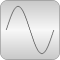# Package Contents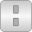Vectors Library of functions operating on vectors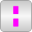BooleanVectors Library of functions operating on Boolean vectors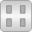Matrices Library of functions operating on matrices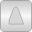Nonlinear Library of functions operating on nonlinear equations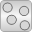Random Library of functions for generating random numbers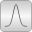Distributions Library of distribution functions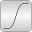Special Library of special mathematical functions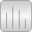FastFourierTransform Library of functions for the Fast Fourier Transform (FFT)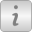Icons Icons for Math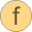isEqual Determine if two Real scalars are numerically identicalisPowerOf2 Determine if the integer input is a power of 2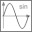sin Sine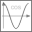cos Cosine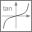tan Tangent (u shall not be -pi/2, pi/2, 3*pi/2, ...)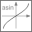asin Inverse sine (-1 <= u <= 1)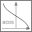acos Inverse cosine (-1 <= u <= 1)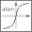atan Inverse tangent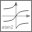atan2 Four quadrant inverse tangent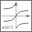atan3 Four quadrant inverse tangent (select solution that is closest to given angle y0)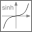sinh Hyperbolic sine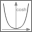cosh Hyperbolic cosine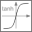tanh Hyperbolic tangent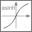asinh Inverse of sinh (area hyperbolic sine)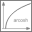acosh Inverse of cosh (area hyperbolic cosine)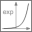exp Exponential, base e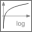log Natural (base e) logarithm (u shall be > 0)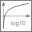log10 Base 10 logarithm (u shall be > 0)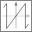wrapAngle Wrap angle to interval ]-pi,pi] or [0,2*pi[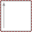baseIcon1 This icon will be removed in future Modelica versions, use Modelica.Math.Icons.AxisLeft instead.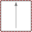baseIcon2 This icon will be removed in future Modelica versions, use Modelica.Math.Icons.AxisCenter instead.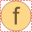tempInterpol1 Temporary function for linear interpolation (will be removed)tempInterpol2 Temporary function for vectorized linear interpolation (will be removed)

# Information

This information is part of the Modelica Standard Library maintained by the Modelica Association.

This package contains basic mathematical functions (such as sin(..)), as well as functions operating on vectors, matrices, nonlinear functions, and Boolean vectors.

#### Main Authors

Martin Otter and Marcus Baur
Deutsches Zentrum für Luft- und Raumfahrt e.V. (DLR)
Institut für Systemdynamik und Regelungstechnik (DLR-SR)
Forschungszentrum Oberpfaffenhofen
D-82234 Wessling
Germany
email: Martin.Otter@dlr.de